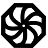# Programming-Idioms# 200
Implementation
JS

Be concise.

Be useful.

All contributions dictatorially edited by webmasters to match personal tastes.

Please try to avoid dependencies to third-party libraries and frameworks.

Other implementations
`math`
`h := hypot(x,y);`
`include Math`
`h = hypot(x, y)`
`import math`
`h = math.hypot(x, y)`
`h = hypot(x,y)`
`use Math::AnyNum qw(hypot);`
`my \$h = hypot \$x, \$y;`
```\$h = hypot(\$x, \$y)

// before PHP 4.1
\$h = sqrt(\$x*\$x + \$y*\$y);
```
`import :math`
```def sq(x) do
x*x
end

def hypo(a,b) do
sqrt(sq(a) + sq(b))
end```
`import "math"`
`h := math.Hypot(x, y)`
```fn hypot(x:f64, y:f64)-> f64 {
let num = x.powi(2) + y.powi(2);
num.powf(0.5)
}```